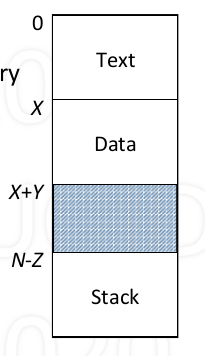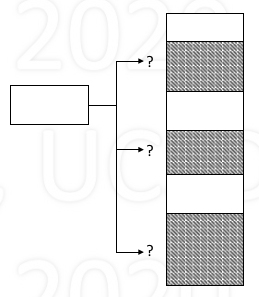0%

## Basic

1. Allocation of memory occurs when

• new process is created
• process requests more memory
2. Freeing of memory occurs when

• process exits
• process no longer needs memory it requested
3. Memory overview

• Porcess memory store:

• Text: code of program
• Data: static varibales, heap
• Stack: automatic variables, activation records
• Other: shared memory regions
• Process memory address space• Address space

• set of addresses to access memory
• Typically, linear and sequential
• 0 to N-1
• Example (right):

• Text of size X at 0 ~ X-1
• Data of size Y at X ~ X+Y-1
• Stack of size Z at N-Z ~ N-1(grow reversely)
• Compiler’s model of memory

• Compiler generates memory address

• Address ranges of text, data, stack
• allow data and stack to grow
• What is not known in compiler

• Physical memory size
• Allocated region of physical memory
• Memory characteristics

• Size, fixed or variable (max size)
• Permission: r, w, x
4. Goal: support multiple processes

• Support programs running “simultaneously”

• implement process abstraction
• Multiplex CPU time over all runnable processes
• Disk r/w speed is low: must keep multiple processes in memory
• Process requires more than CPU time: memory

5. Memory issues and topics

• Where should process memories be placed? -> Memory Management
• How does the compiler model memory? -> Logical memory model, segmentation
• How to deal with limited physical memory? -> Virtual memory, paging
• Machanism and Policies

## Memory Managemeng Implementation

1. example process• Phsical memory starts as one empty “hole”
• When processes are created, areas get allocated
• To allocate memory

• find large enough hole
• allocate region within hole
• mostly leaves smaller hole (when the hole size does not exactly match the size of process memory)
• when process exit (or memeory no longer needed), release that area, which create a new hole, coaleasce with adjacent
• problem: if there are multiple holes, which to select?

• First fit: simple, fast

• consider tradeoff: fit vs. search time
• memory is cheap, time is expensive
• Best fit: optimal, must check every hole, leaves very small fragments

• Worst fit: leaves large fragments, must check every hole
• complication: is region fixed or variable size?

2. Fragmentation

• Over time, memory becomes fragmented, there may be signficant unused space (fragmented)
• Internal fragmentation

• Unused space within (allocated) block
• Cannot be allocated to others
• Can come in handy for growth (for stack or heap)
• External fragmentation

• Unused space outside any blocks (holes)
• Can be allocated (too small/not useful)
• Approaches

• Compaction

• Simple idea
• Very time consuming
• Break block (to be allocated) into sub-blocks

• Easier to fit
• But complex
• Use pre-sized holes

• Same-sized holes:

• all holes same, easy allocation
• may be too small which is inflexible
• Varaiety of sizes (small, medium, large)

• more flexible
• complex
• what should sizes be? how to determine
• Not adaptable, cause internel fragmentation

3. Some rules

• 50% Rule: m = n / 2

• Block: an allocated block
• Hole: free space between blocks
• m = number of holes
• n = number of blocks
• Unused Memory Rule: f = k / (k + 2)

• Given average size of blocks and holes are known
• b = average size of blocks
• h = average size of holes
• k = h / b, ratio of average hole-to-block size
• f = k / (k + 2) is fraction space lost to holes
• Usage:

• k = 1, f = 1/3 -> avg hole size = avg block size, 33% waste
• k = 2, f = 1/2 -> avg hole size = 2 * avg block size, 50% waste
• k = 8, f = 4/5 -> avg hole size = 8 * avg block size, 80% waste
• Limits

• In general, f increases with increasing k

• as $k \rightarrow \infty, f \rightarrow 1$
• Alternatively, f decreases with decreasing k

• as $k \rightarrow 0, f \rightarrow 0$
4. The Buddy System

• Partition into power-of-2 size chunks
• Allocation: given request for size r

• Free: free the chunk and coalesce with buddy

• visualization

• Data structure for buddy-system -> binary tree